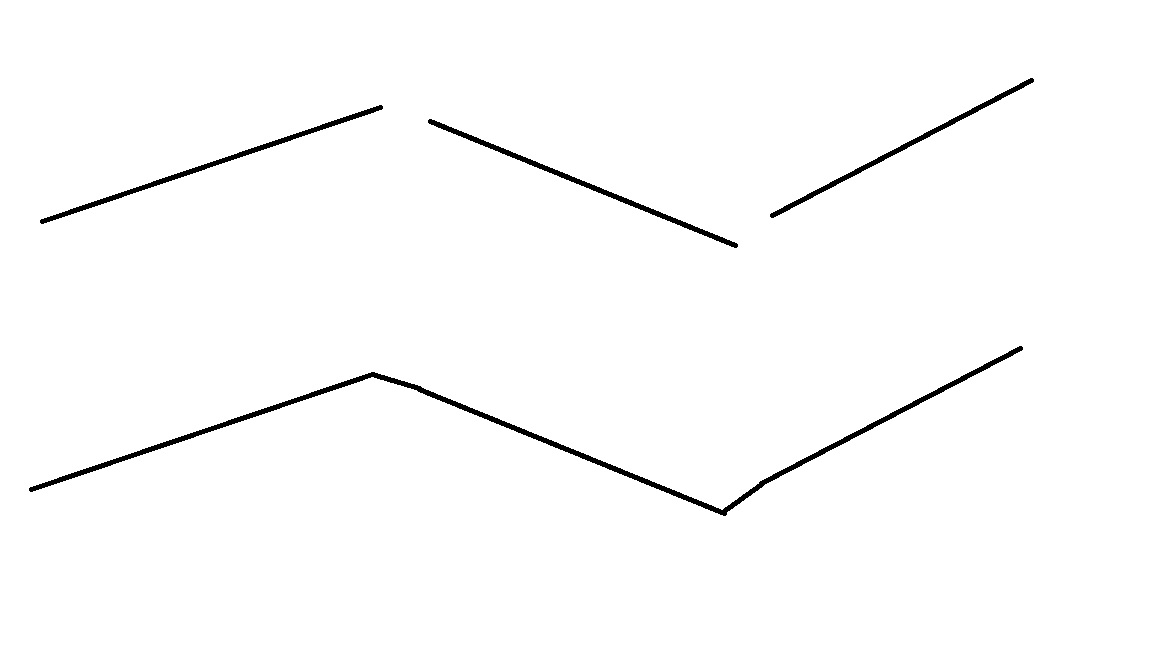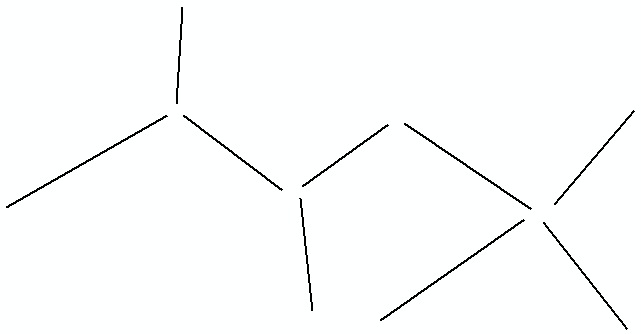# Connecting Discontinuous Line Segments

4816
3
Jump to solution
01-28-2015 01:52 PMNew Contributor

I have a shape file with a series of line segments that are discontinuous, I want to join them together so they form a continuous line based on a common attribute from the attribute table. I already understand how to merge the lines using Merge in the editing tool bar, but I'm not clear on if there is a way to get those segments to link up as shown in the image below (I have the broken lines on top, I want the continuous line below) without manually snapping them together point by point, as it is a large file with numerous such segments. Is there a tool in ArcGIS that can do this? Thanks.  (Note: I've seen some other postings mention the Dissolve tool, but I don't see how it applies here).1 Solution

Accepted SolutionsbyEsri Esteemed Contributor

Hi Daniel Walters‌,

I saw your question and thought, let's give it a try...

Based on this input:Is creates this output:Using this code:

`import mathdef main():    import arcpy    fc_in = r"D:\Xander\GeoNet\Nueva carpeta\test.gdb\lines"    fc_out = r"D:\Xander\GeoNet\Nueva carpeta\test.gdb\lines_out"    tolerance = 150 # max distance to snap to a node    # create dicts    dct_lines = getPolylineDict(fc_in)    dct_from, dct_to = getFromAndToNodes(dct_lines)    # loop through polylines    for oid, polyline in dct_lines.items():        # search candidates from point        pnt_from = dct_from[oid]        dist_min = tolerance + 0.01        pnt_found = None        for oid_from, pnt in dct_from.items():            if oid_from != oid:                dist = calcDistance(pnt_from, pnt)                if dist < dist_min:                    dist_min = dist                    pnt_found = arcpy.Point(pnt.X, pnt.Y)        for oid_to, pnt in dct_to.items():            if oid_to != oid:                dist = calcDistance(pnt_from, pnt)                if dist < dist_min:                    dist_min = dist                    pnt_found = arcpy.Point(pnt.X, pnt.Y)        # change line from point        if pnt_found != None:            polyline = insertPointAtStart(polyline, pnt_found)            dct_lines[oid] = polyline            dct_from, dct_to = getFromAndToNodes(dct_lines)        # search candidates to points        pnt_to = dct_to[oid]        dist_min = tolerance + 0.01        pnt_found = None        for oid_from, pnt in dct_from.items():            if oid_from != oid:                dist = calcDistance(pnt_to, pnt)                if dist < dist_min:                    dist_min = dist                    pnt_found = arcpy.Point(pnt.X, pnt.Y)        for oid_to, pnt in dct_to.items():            if oid_to != oid:                dist = calcDistance(pnt_to, pnt)                if dist < dist_min:                    dist_min = dist                    pnt_found = arcpy.Point(pnt.X, pnt.Y)        # change line end point        if pnt_found != None:            polyline = addPointAtEnd(polyline, pnt_found)            dct_lines[oid] = polyline            dct_from, dct_to = getFromAndToNodes(dct_lines)    # create list of polylines    lst_polylines = dct_lines.values()    arcpy.CopyFeatures_management(lst_polylines, fc_out)def getPolylineDict(fc_in):    dct = {}    flds = ("OID@", "SHAPE@")    with arcpy.da.SearchCursor(fc_in, flds) as curs:        for row in curs:            dct[row] = row    del row, curs    return dctdef getFromAndToNodes(dct):    dct_from = {}    dct_to = {}    for oid, polyline in dct.items():        dct_from[oid] = polyline.firstPoint        dct_to[oid] = polyline.lastPoint    return dct_from, dct_todef insertPointAtStart(polyline, pnt_found):    sr = polyline.spatialReference    lst_pnts = [pnt_found]    for part in polyline:        for pnt in part:            lst_pnts.append(pnt)    return arcpy.Polyline(arcpy.Array(lst_pnts), sr)def addPointAtEnd(polyline, pnt_found):    sr = polyline.spatialReference    lst_pnts = []    for part in polyline:        for pnt in part:            lst_pnts.append(pnt)    lst_pnts.append(pnt_found)    return arcpy.Polyline(arcpy.Array(lst_pnts), sr)def calcDistance(pnt_to, pnt):    return math.hypot(pnt_to.X - pnt.X, pnt_to.Y - pnt.Y)if __name__ == '__main__':    main()`

If you have any questions, just let me know...

Kind regards, Xander

3 RepliesbyEsri Esteemed Contributor

Hi Daniel Walters‌,

I saw your question and thought, let's give it a try...

Based on this input:Is creates this output:Using this code:

`import mathdef main():    import arcpy    fc_in = r"D:\Xander\GeoNet\Nueva carpeta\test.gdb\lines"    fc_out = r"D:\Xander\GeoNet\Nueva carpeta\test.gdb\lines_out"    tolerance = 150 # max distance to snap to a node    # create dicts    dct_lines = getPolylineDict(fc_in)    dct_from, dct_to = getFromAndToNodes(dct_lines)    # loop through polylines    for oid, polyline in dct_lines.items():        # search candidates from point        pnt_from = dct_from[oid]        dist_min = tolerance + 0.01        pnt_found = None        for oid_from, pnt in dct_from.items():            if oid_from != oid:                dist = calcDistance(pnt_from, pnt)                if dist < dist_min:                    dist_min = dist                    pnt_found = arcpy.Point(pnt.X, pnt.Y)        for oid_to, pnt in dct_to.items():            if oid_to != oid:                dist = calcDistance(pnt_from, pnt)                if dist < dist_min:                    dist_min = dist                    pnt_found = arcpy.Point(pnt.X, pnt.Y)        # change line from point        if pnt_found != None:            polyline = insertPointAtStart(polyline, pnt_found)            dct_lines[oid] = polyline            dct_from, dct_to = getFromAndToNodes(dct_lines)        # search candidates to points        pnt_to = dct_to[oid]        dist_min = tolerance + 0.01        pnt_found = None        for oid_from, pnt in dct_from.items():            if oid_from != oid:                dist = calcDistance(pnt_to, pnt)                if dist < dist_min:                    dist_min = dist                    pnt_found = arcpy.Point(pnt.X, pnt.Y)        for oid_to, pnt in dct_to.items():            if oid_to != oid:                dist = calcDistance(pnt_to, pnt)                if dist < dist_min:                    dist_min = dist                    pnt_found = arcpy.Point(pnt.X, pnt.Y)        # change line end point        if pnt_found != None:            polyline = addPointAtEnd(polyline, pnt_found)            dct_lines[oid] = polyline            dct_from, dct_to = getFromAndToNodes(dct_lines)    # create list of polylines    lst_polylines = dct_lines.values()    arcpy.CopyFeatures_management(lst_polylines, fc_out)def getPolylineDict(fc_in):    dct = {}    flds = ("OID@", "SHAPE@")    with arcpy.da.SearchCursor(fc_in, flds) as curs:        for row in curs:            dct[row] = row    del row, curs    return dctdef getFromAndToNodes(dct):    dct_from = {}    dct_to = {}    for oid, polyline in dct.items():        dct_from[oid] = polyline.firstPoint        dct_to[oid] = polyline.lastPoint    return dct_from, dct_todef insertPointAtStart(polyline, pnt_found):    sr = polyline.spatialReference    lst_pnts = [pnt_found]    for part in polyline:        for pnt in part:            lst_pnts.append(pnt)    return arcpy.Polyline(arcpy.Array(lst_pnts), sr)def addPointAtEnd(polyline, pnt_found):    sr = polyline.spatialReference    lst_pnts = []    for part in polyline:        for pnt in part:            lst_pnts.append(pnt)    lst_pnts.append(pnt_found)    return arcpy.Polyline(arcpy.Array(lst_pnts), sr)def calcDistance(pnt_to, pnt):    return math.hypot(pnt_to.X - pnt.X, pnt_to.Y - pnt.Y)if __name__ == '__main__':    main()`

If you have any questions, just let me know...

Kind regards, XanderNew Contributor

Hi XanderBarkker,

Thanks a lot for your python script. It helped me a lot with a project am working on. I am running the script on a file and currently having some error. Please see below:Any help or input is welcomes. Thanks!Regular Contributor

nice job with python coding by Xander,

Also, nice to use the simple generalization tool called 'Extend Line' at ArcGIS Help (10.2, 10.2.1, and 10.2.2)

The following simple standalone is copied from the Help

`# Name: ExtendLine.py# Description:  Clean up street centerlines that were digitized without #                      having set proper snapping environments# import system modules import arcpyfrom arcpy import env# Set environment settingsenv.workspace = "C:/data"# Make backup copy of streets feature class, since modification with #  the Editing tools below is permanentstreets = "majorrds.shp"streetsBackup = "C:/output/Output.gdb/streetsBackup"arcpy.CopyFeatures_management(streets, streetsBackup)# Trim street lines to clean up danglesarcpy.TrimLine_edit(streets, "10 Feet", "KEEP_SHORT")# Extend street lines to clean up danglesarcpy.ExtendLine_edit(streets, "15 Feet", "EXTENSION")`